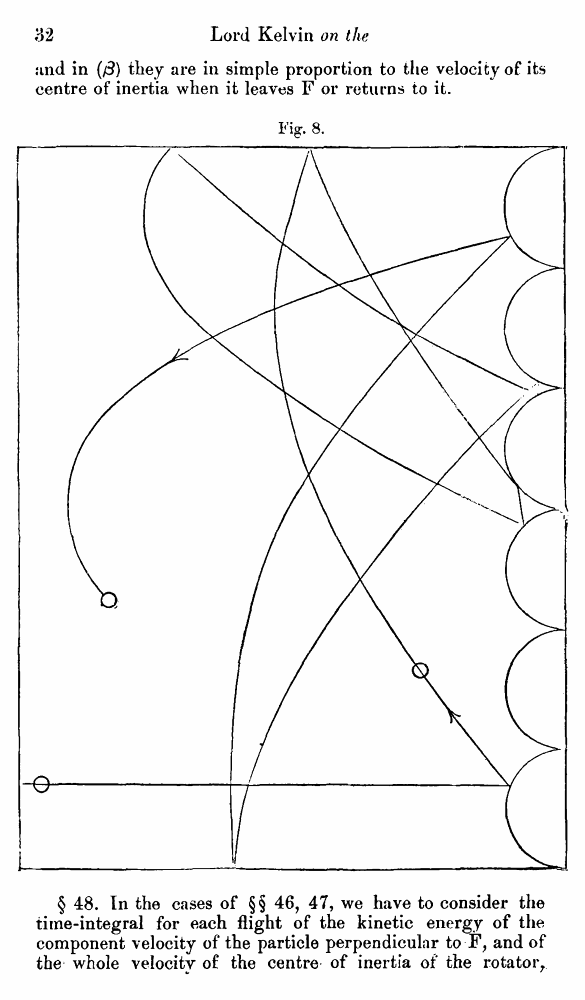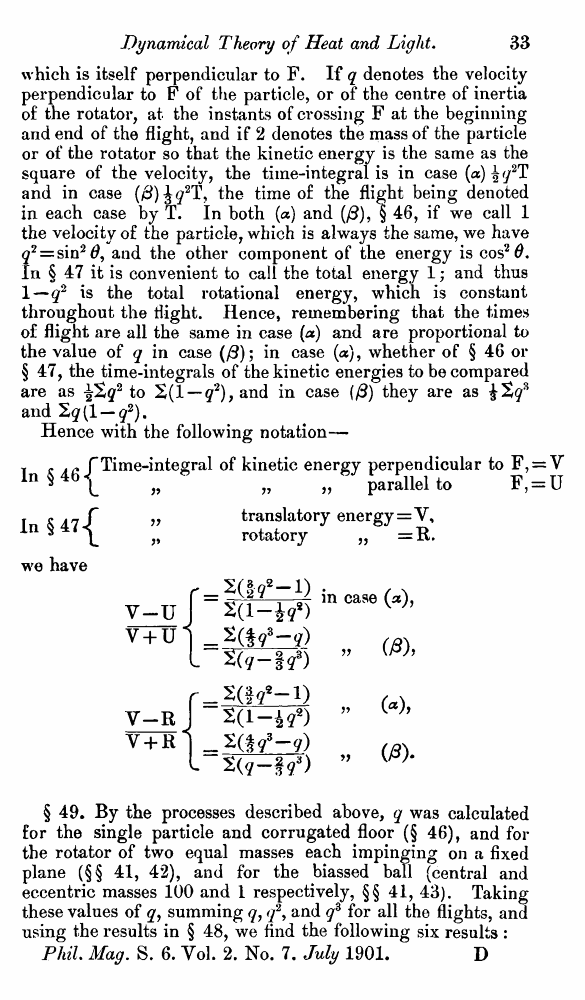# Lord Kelvin. Nineteenth-Century Clouds over the Dynamical Theory of Heat and Light. // Phil. Mag. S. 6. Vol. 2. No. 7. July 1901.

В начало   Другие форматы   <<<     Страница 32   >>>1  2  3  4  5  6  7  8  9  10  11  12  13  14  15  16  17  18  19  20  21  22  23  24  25  26  27  28  29  30  31  32 33  34  35  36  37  38  39  40 iind in (/3) they are in simple proportion to the velocity of its centre of inertia when it leaves F or returns to it. Fig:. 8. § 48. In the cases of §§ 46, 47, we have to consider the time-integral for each flight of the kinetic energy of the component velocity of the particle perpendicular to F, and of the whole velocity of the centre of inertia of the rotator, which is itself perpendicular to F. If q denotes the velocity perpendicular to F of the particle, or of the centre of inertia of the rotator, at the instants of crossing F at the beginning and end of the flight, and if 2 denotes the mass of the particle or of the rotator so that the kinetic energy is the same as the square of the velocity, the time-integral is in case (a) ^q2T and in case (#) the time of the flight being denoted in each case by T. In both (a) and (/3), § 46, if we call 1 the velocity of the particle, which is always the same, we have q2 = sin2#, and the other component of the energy is cos2#. In § 47 it is convenient to call the total energy 1; and thus 1—q2 is the total rotational energy, which is constant throughout the flight. Hence, remembering that the times of flight are all the same in case (a) and are proportional to the value of q in case (/3); in case (a), whether of § 46 or § 47, the time-integrals of the kinetic energies to be compared are as ^2g2 to 2(1 — q2), and in case (/3) they are as £2